Actions

(diff) ← Older revision | Latest revision (diff) | Newer revision → (diff)

A quadratic form in two variables, i.e. having the form(*)

If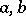andare integers, the binary quadratic form is said to be integral. The expression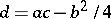is called the discriminant or determinant of the binary quadratic form. The expressionis also sometimes referred to as the discriminant. The arithmetic theory of binary quadratic forms originated with P. Fermat, who proved that any prime number of the form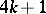can be represented as the sum of two squares of integers. The theory of quadratic forms was completed by J.L. Lagrange and by C.F. Gauss. This theory is a special case of the theory of quadratic forms invariables; the arithmetic theory of binary quadratic forms is equivalent to the theory of ideals in quadratic fields, and is one of the origins of algebraic number theory (cf. Quadratic form; Quadratic field).

The number of genera of binary quadratic forms with discriminant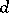equals, whereis the number of different prime divisors of, except for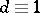(),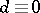(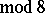), whenis increased by one; if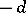is a square, the number of different binary quadratic forms is doubled. The number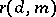of essentially different primitive representations of a numberby a complete system of binary quadratic forms with discriminantis equal to the number of solutions of the equation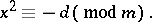As in the general case, there exists an algorithm which reduces the problem of solving a given second-degree Diophantine equation in two unknowns (in particular, an equation) to the problem on the arithmetic equivalence of two binary quadratic forms.

All integral automorphisms of a primitive formwithcan be represented in the form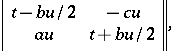where, andandare integers (cf. Pell equation). Therefore, the problem on the equivalence of two forms is solved by the reduction theory of binary quadratic forms. The reduction theory of positive-definite binary quadratic forms is a special case of the reduction theory of positive-definite quadratic forms according to H. Minkowski. The reduction theory of integral indefinite binary quadratic forms can be reduced to the reduction theory of quadratic irrationalities (, ).

An important role in the theory of numbers is played by the arithmetic function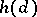— the number of classes of primitive integral binary quadratic forms with determinant. It is known that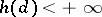. Some idea of the rate of increase of the functioncan be obtained from Siegel's theorem: Let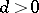, then for anythere exist constantsand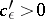which satisfy the condition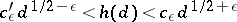(a similar formula is also valid for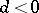).

Letbe an integer,or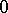(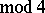), suppose that ifthen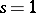or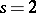, and letbe the quadratic field which is obtained by adjoining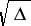to the field of rational numbers. A correspondence has been established between the integral idealsof the field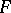and the integral quadratic forms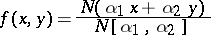withas determinant. This results in a one-to-one correspondence (up to a transition to conjugate classes of ideals) between the ideal classes of the fieldand the classes of binary quadratic forms. In this correspondence, multiplication of ideal classes defines a composition of classes of binary quadratic forms.

As in the case of forms invariables, the theory of binary quadratic forms can be generalized to include forms (*) with coefficientsandin a given algebraic number field.

There are various variants in the definitions of an integral form, the discriminant of the form, equivalence of forms, and the classes and the genera of forms. The definition of integral forms given above is due to L. Kronecker. Gauss  stipulated that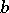be even. In determining the equivalence (and the class of forms), only the substitutions with discriminantmay be considered; in other cases discriminants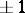are considered. The definition of a genus given in  is wider than that given by Gauss.

How to Cite This Entry: